Most Affordable JEE | NEET | 8,9,10 Preparation by Kota's Top IITian Doctor Faculties

# Aromatic Compounds - JEE Advanced Previous Year Questions with Solutions`
JEE Advanced Previous Year Questions of Chemistry with Solutions are available at eSaral. Practicing JEE Advanced Previous Year Papers Questions of Chemistry will help the JEE aspirants in realizing the question pattern as well as help in analyzing weak & strong areas. Simulator   Previous Years JEE Advance Questions
Q. In the reaction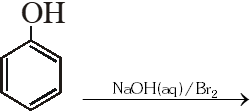the intermediate(s) is(are) –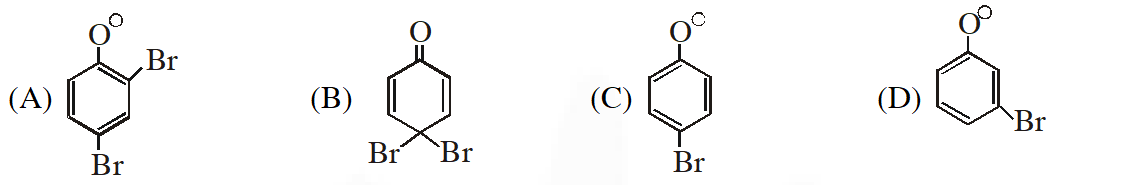[JEE 2009]
Ans. (A,B,C)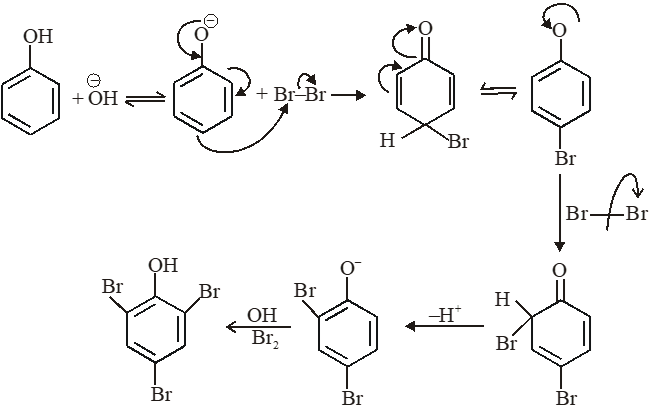Q. The compounds P, Q and S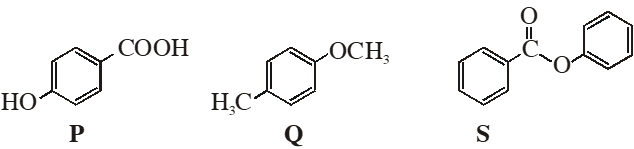were separately subjected to nitration using $\mathrm{HNO}_{3} / \mathrm{H}_{2} \mathrm{SO}_{4}$ mixture. The product formed in each case respectively, is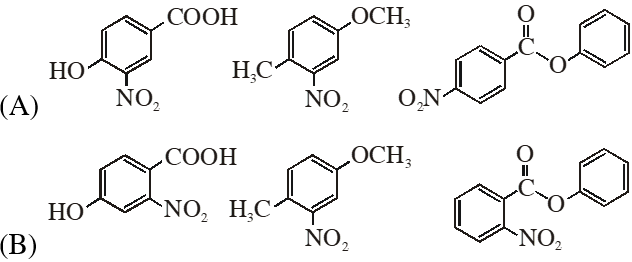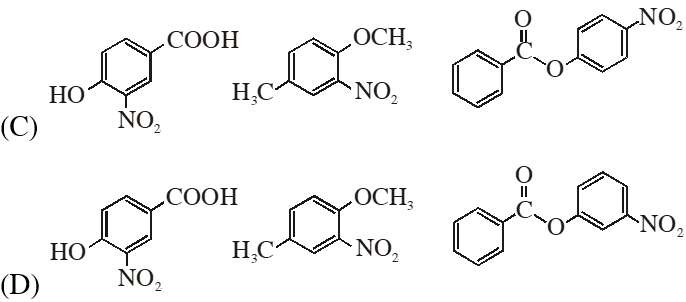[JEE 2009]
Ans. (C) (1)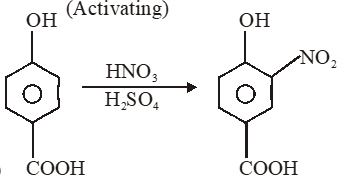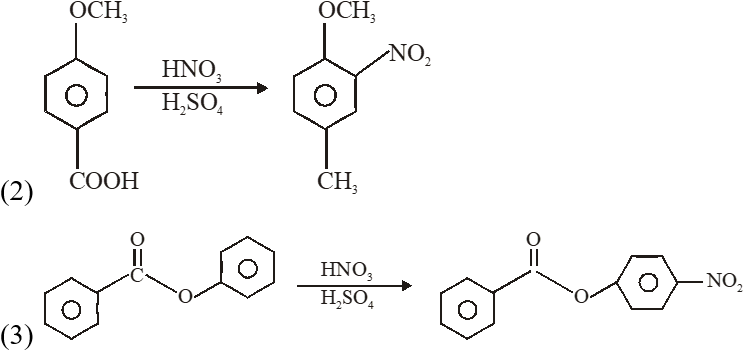Q. The correct acidity order of the following is :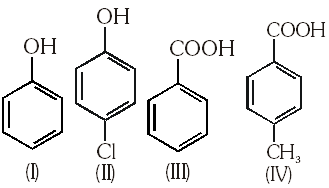(A) (III) > (IV) > (II) > (I) (B) (IV) > (III) > (I) > (II) (C) (III) > (II) > (I) > (IV) (D) (II) > (III) > (IV) > (I) [JEE 2009]
Ans. (A)
Q. In the following carbocation, $\mathrm{H} / \mathrm{CH}_{3}$ that is most likely to migrate to the positively charged carbon is :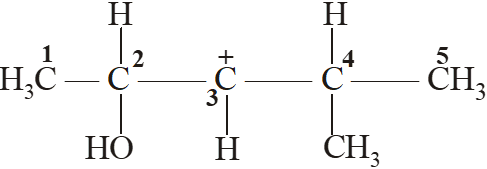(A) $\mathrm{CH}_{3}$ at $\mathrm{C}-4$ (B) H at C – 4 (C) $\mathrm{CH}_{3}$ at $\mathrm{C}-2$ (D) H at C - 2 [JEE 2009]
Ans. (D)
Q.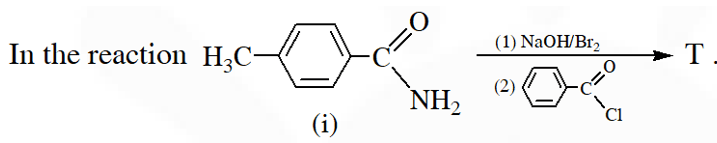The structure of the Product T is :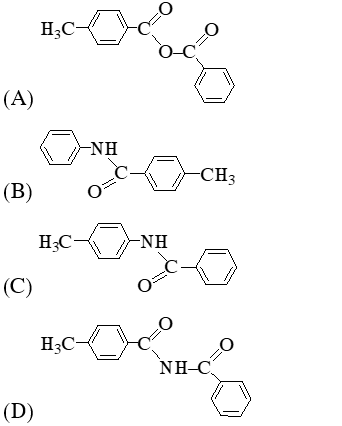[JEE 2010]
Ans. (C)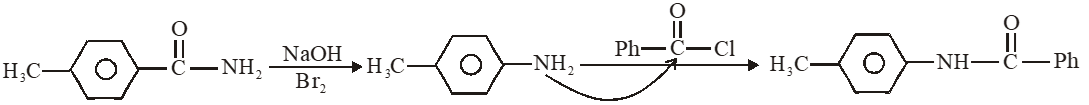Q. Among the following compounds, the most acidic is (A) p-nitrophenol (B) p-hydroxybenzoic acid (C) o-hydroxybenzoic acid (D) p-toluic acid [JEE 2011]
Ans. (C)
Q. The maximum number of isomers (including stereoisomers) that are possible on mono-chlorination of the following compounds, is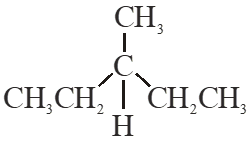[JEE 2011]
Ans. 8
Q. Among P, Q, R and S, the aromatic compound(s) is / are :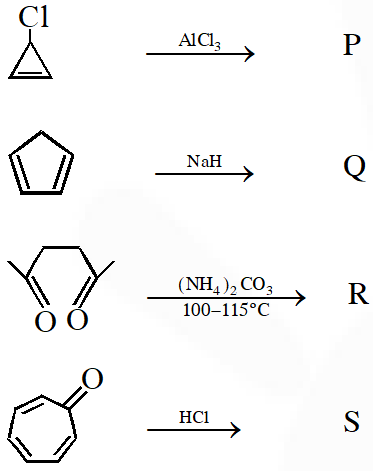(A) P (B) Q (C) R (D) S [JEE 2013]
Ans. (A,B,C,D)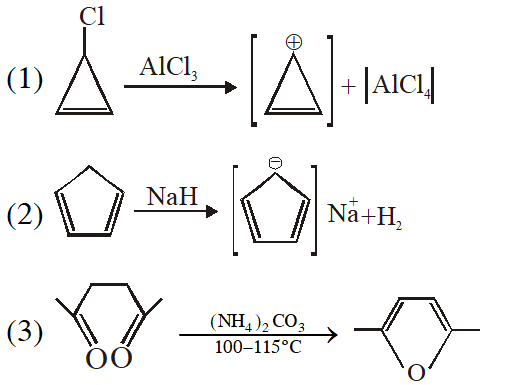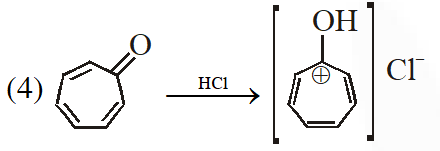Q. The major product(s) of the following reaction is (are) –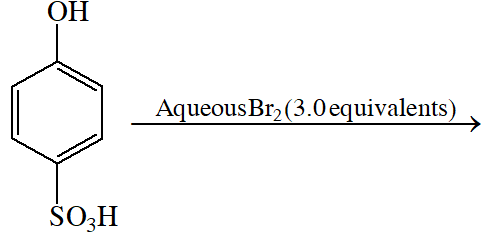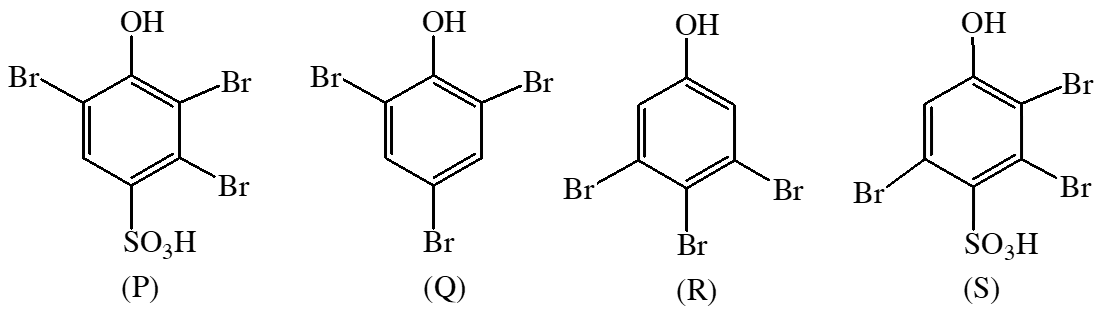(A) P (B) Q (C) R (D) S [JEE 2013]
Ans. (B)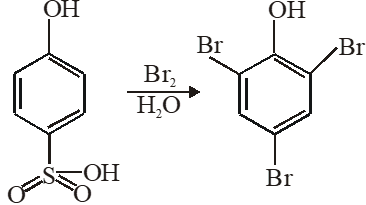Q. In the following reaction, the product (s) formed is (are)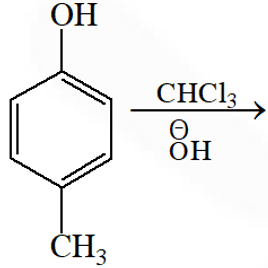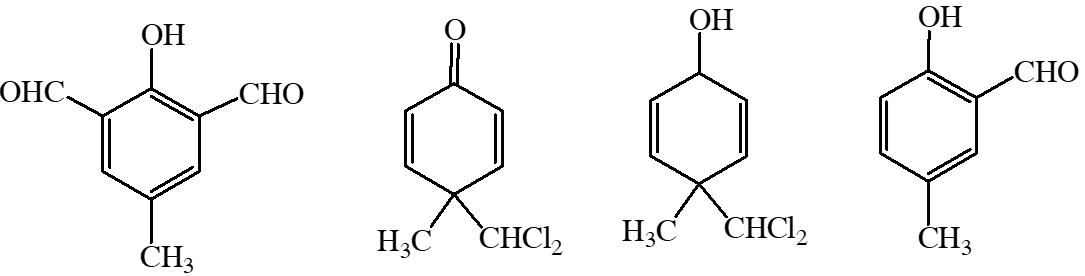(A) P (major) (B) Q (minor) (C) R (minor) (D) S (major) [JEE 2013]
Ans. (B,D)
Q. In the following reaction sequences V and W are , respectively –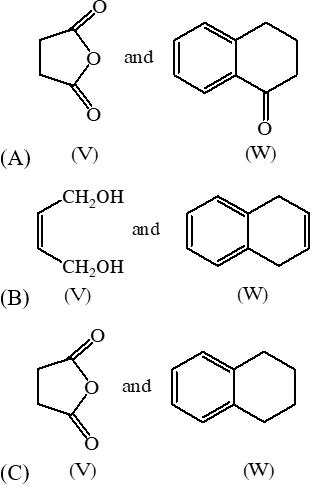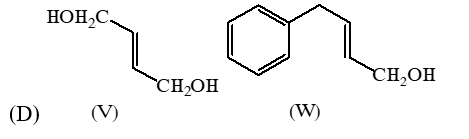[JEE 2013]
Ans. (A)
Paragraph for Question 11 and 12 P & Q are isomers of dicarboxylic acid $\mathrm{C}_{4} \mathrm{H}_{4} \mathrm{O}_{4}$. Both decolourize $\mathrm{Br}_{2} / \mathrm{H}_{2} \mathrm{O}$, On heating P forms the cyclic anhydride. Upon treatment with dilute alkaline $\mathrm{KMnO}_{4}$, P as well as Q could produce one or more than one from S, T and U. [JEE 2013]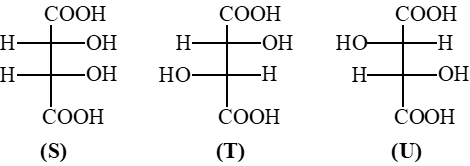Q. Compounds formed from P and Q are respectively (A) Optically active S and optically active pair (T, U) (B) Optically inactive S and optically inactive pair (T, U) (C) Optically active pair (T, U) and optically active S (D) Optically inactive pair (T, U) and optically inactive S [JEE 2013]
Ans. (B)
Q. In the reaction shown below, the major product(s) formed is / are :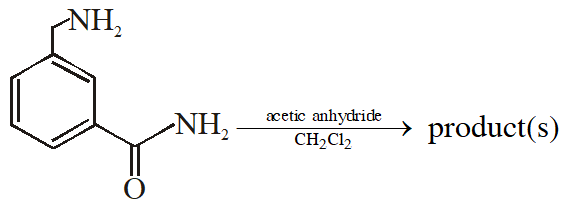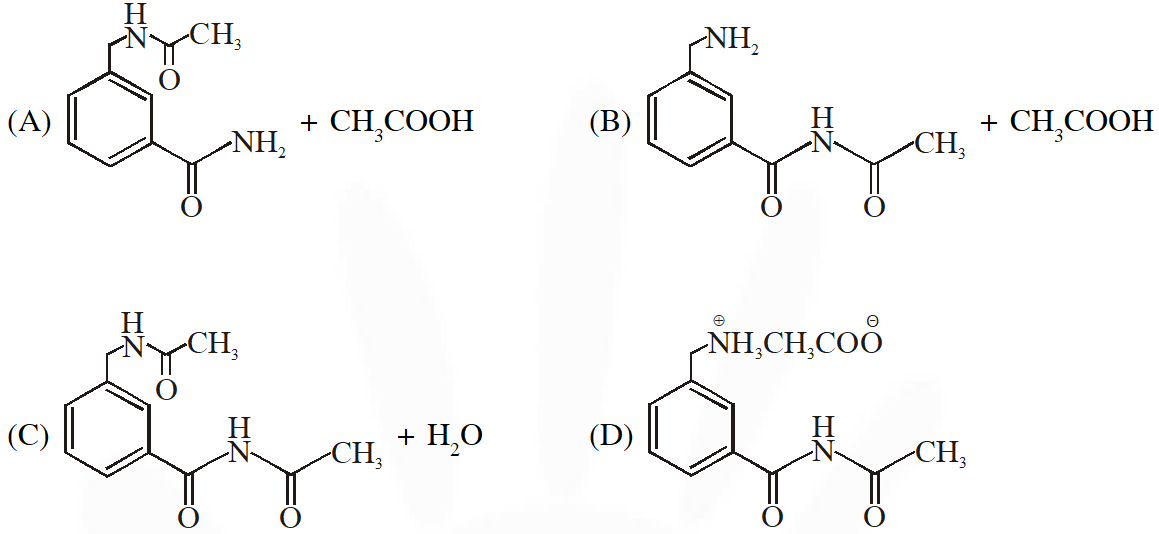[JEE 2014]
Ans. (A)
Q. The reactivity of compound Z with different halogens under appropriate conditions is given below-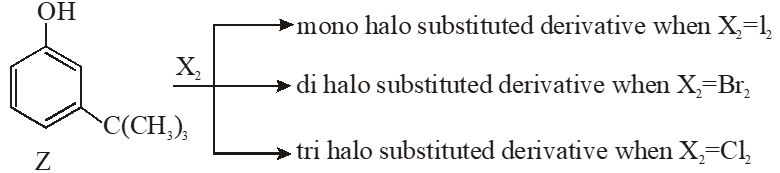The observed pattern of electrop hilic substitution can be explained by – (A) The steric effect of the halogen (B) The steric effect of the tert-butyl group (C) The electronic effect of the phenolic group (D) The electronic effect of the turt-butyl group [JEE 2014]
Ans. (A,B,C)
Q. Match the four starting materials (P , Q, R, S) given in List I with the corresponding reaction scheme (I, II, III, IV) provided in List - II and select the correct answer using the code given below in lists.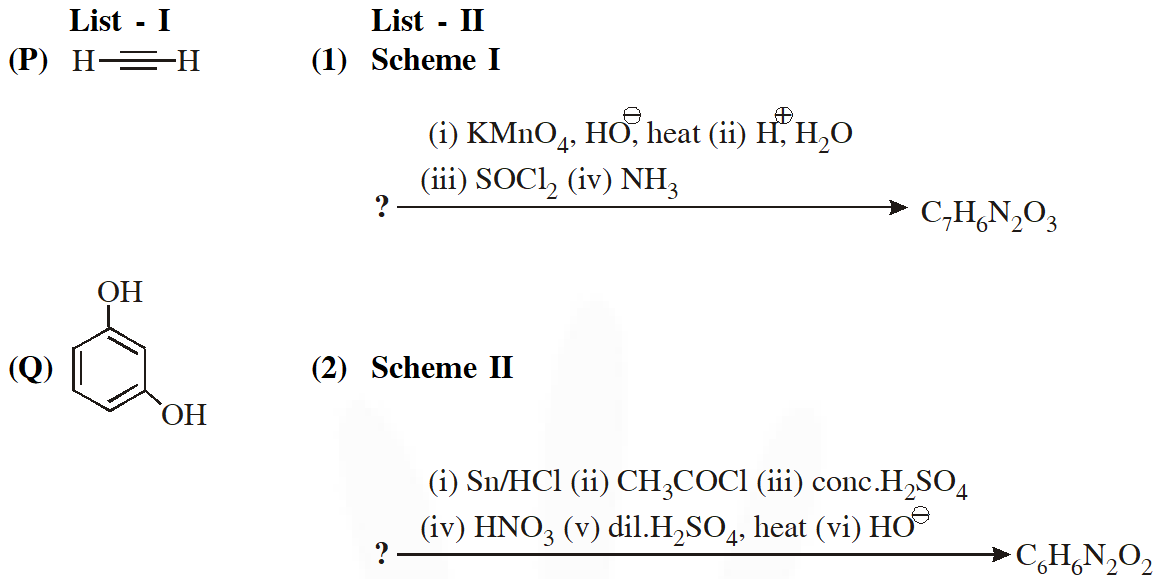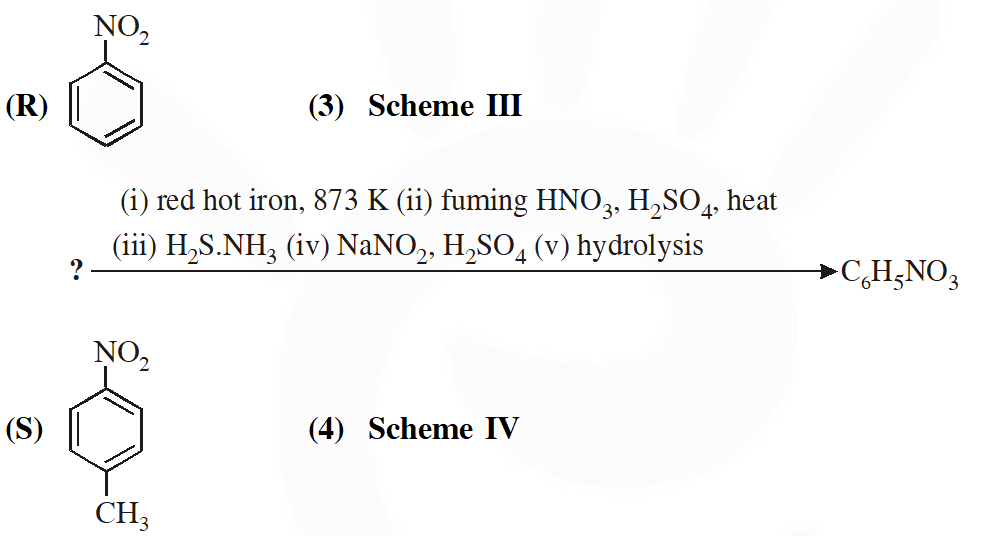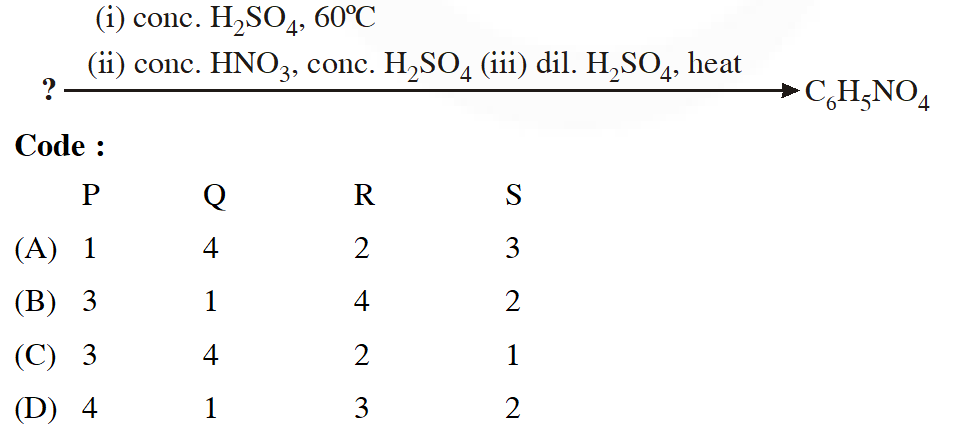[JEE 2014]
Ans. (C)
Q. In the reaction the product E is :-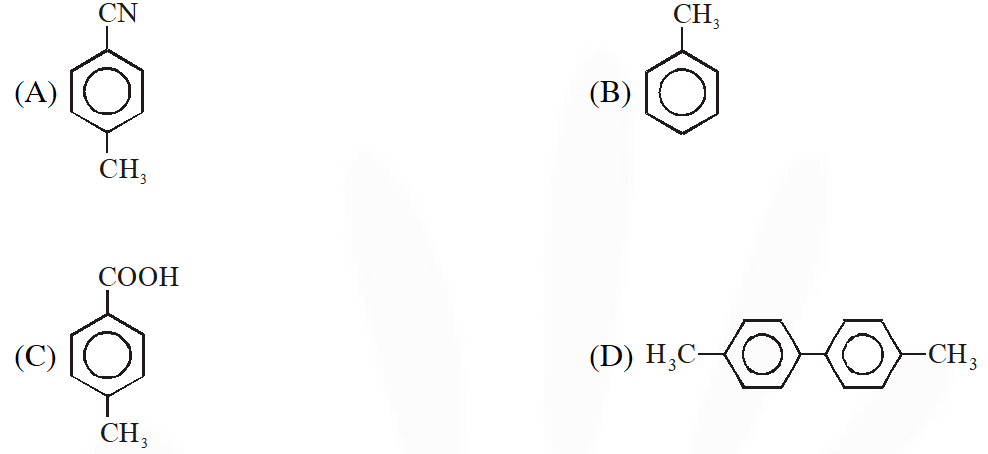[IIT-2015]
Ans. (A)
Q. In the following sequence of reactions :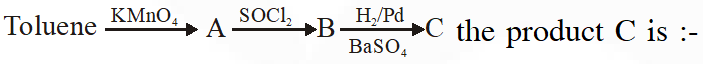(A) $\mathrm{C}_{6} \mathrm{H}_{5} \mathrm{CH}_{2} \mathrm{OH}$ (B) $\mathrm{C}_{6} \mathrm{H}_{5} \mathrm{CHO}$ (C) $\mathrm{C}_{6} \mathrm{H}_{5} \mathrm{COOH}$ (D) $\mathrm{C}_{6} \mathrm{H}_{5} \mathrm{CH}_{3}$ [IIT 2015]
Ans. (B)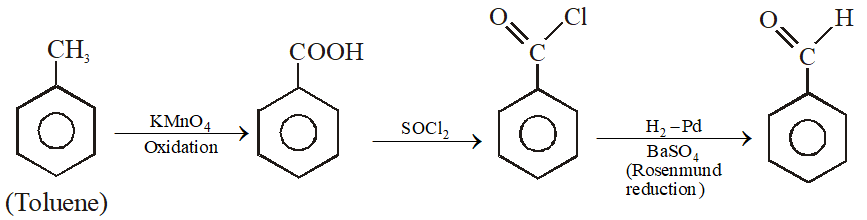Q. The major product U in the following reactions is :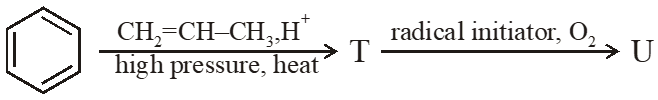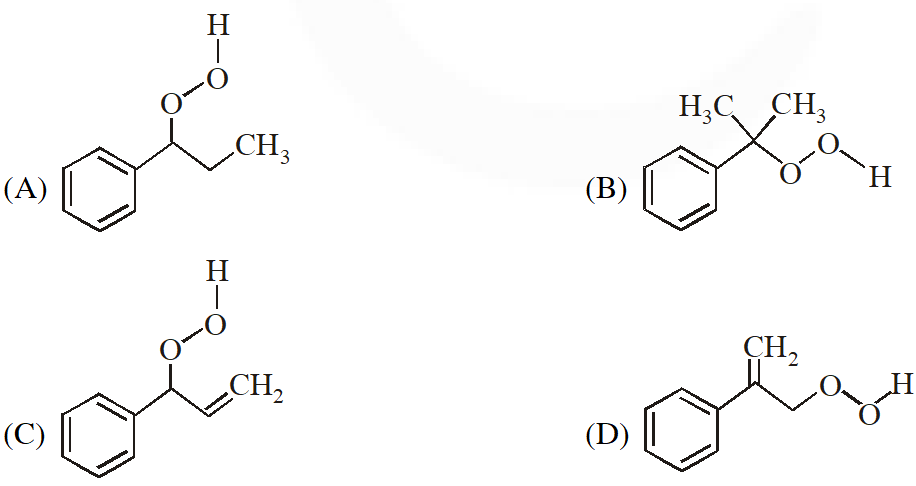[IIT 2015]
Ans. (C)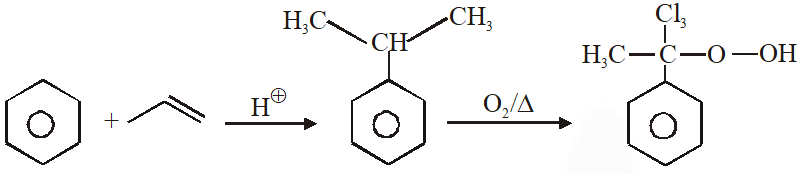Q. In the following reactions, the major product W is :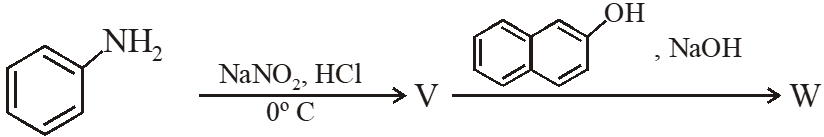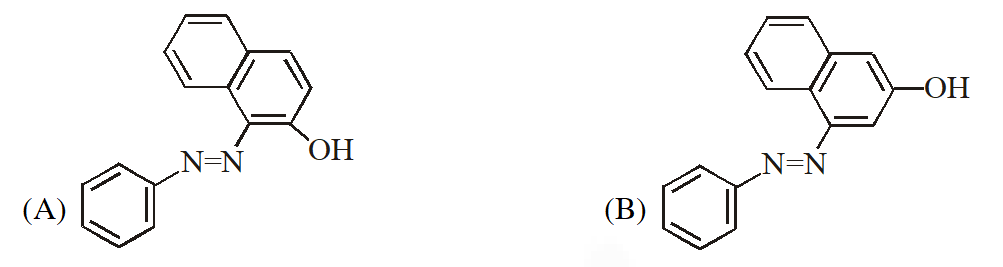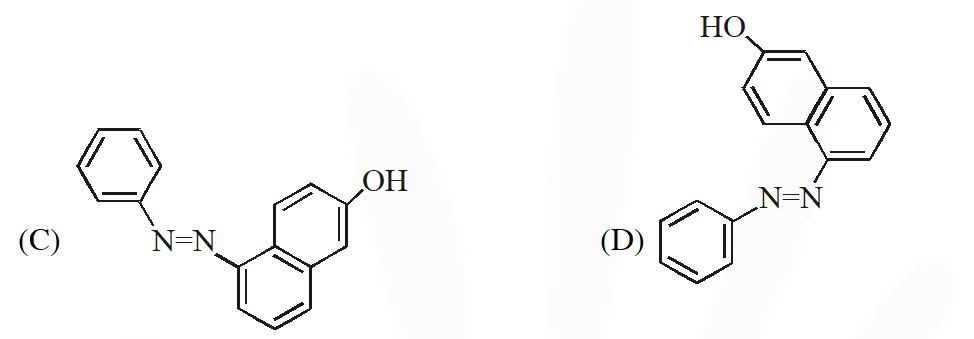[IIT 2015]
Ans. (A)
Q. Among the following the number of reaction(s) that produce(s) benzaldehyde is :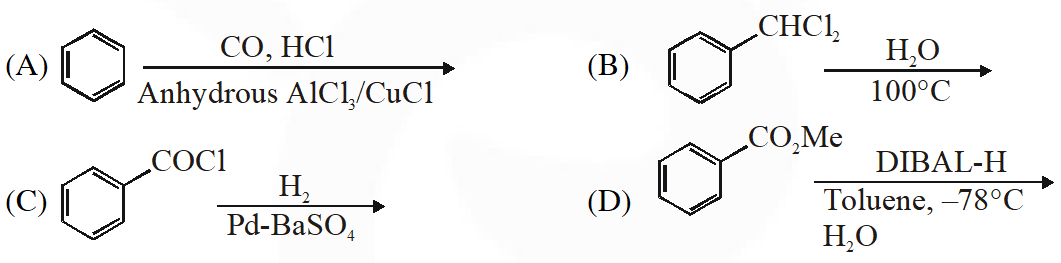[IIT 2015]
Ans. (D)